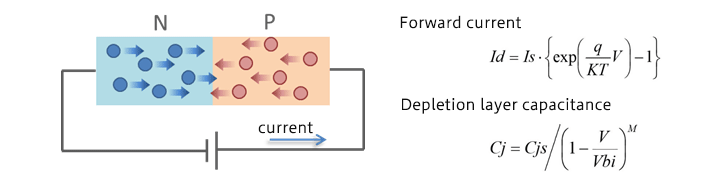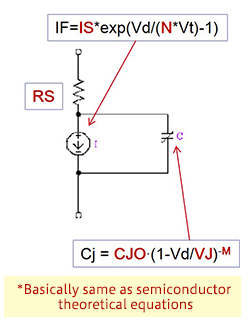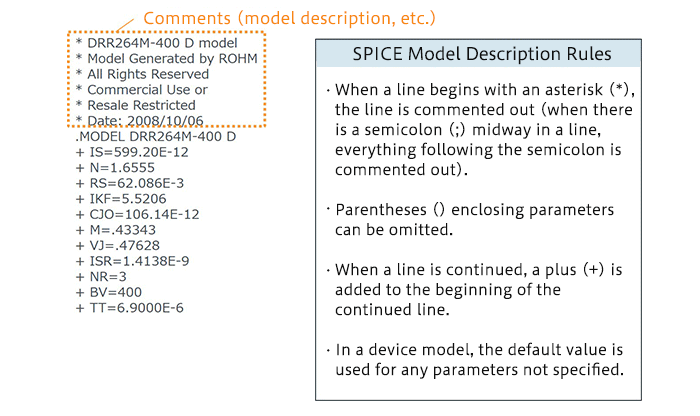Technical Information Site of Power Supply Design

2019.05.09 Simulation

# SPICE Device Models: Diode Example--Part 1

Foundations of Electronic Circuit Simulation

In the previous article it was explained that models used in SPICE simulations can be device models or subcircuit models. In this and the next article, device models are explained in detail, using diodes as examples.

SPICE Device Models: Principle of Operation of Diodes

Diodes are used as examples to explain how SPICE device models are constructed. To this end, we first review the principle of operation of a diode. Here semiconductor theory is involved, but what is important is the parameters used in the device model, and so there is no real need for an understanding of the theory itself.

The diagram below illustrates the basic principle of operation of a silicon diode. When a bias voltage is applied to the diode, the electric field in the depletion layer weakens, carrier diffusion occurs, and a current flows. The forward current Id and the depletion layer capacitance Cj of the diode can be expressed by the following equations.Diode Parameters

The diagram below is the equivalent circuit of a diode. The forward current IF (represented by Id in the equation above), depletion layer capacitance Cj, and parasitic resistance RS are shown. Upon substituting the diode parameters (see the table) into the above equations, we obtain the following model equations.Model equations are essentially the same as semiconductor theoretical equations. In a device model, these parameter values are set.

SPICE Device Models: Case Study of Diode Device Model

A device model of an actual diode is presented. This model is provided by ROHM.Although mentioned in the list of description rules, lines beginning with an asterisk (*) are commented out. Simple explanations of the model and the like can be provided as desired. In this example, the actual description begins with "MODEL". Lines beginning with pluses (+) set parameter values. Please compare the device model description and the parameter table. When a default value can be used as the parameter setting, a value need not be stated.

(Continued to Part 2)

#### Key Points:

・There are two types of SPICE models: “device models” and “subcircuit models”.

・In SPICE device model, each parameter value is set.